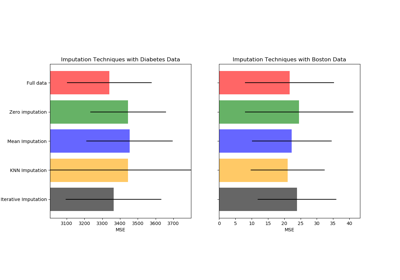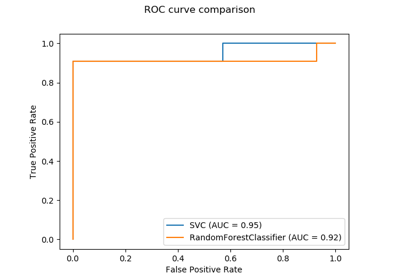# sklearn.impute.KNNImputer¶

class sklearn.impute.KNNImputer(missing_values=nan, n_neighbors=5, weights='uniform', metric='nan_euclidean', copy=True, add_indicator=False)[source]

Imputation for completing missing values using k-Nearest Neighbors.

Each sample’s missing values are imputed using the mean value from n_neighbors nearest neighbors found in the training set. Two samples are close if the features that neither is missing are close.

Read more in the User Guide.

New in version 0.22.

Parameters
missing_valuesnumber, string, np.nan or None, default=np.nan

The placeholder for the missing values. All occurrences of missing_values will be imputed.

n_neighborsint, default=5

Number of neighboring samples to use for imputation.

weights{‘uniform’, ‘distance’} or callable, default=’uniform’

Weight function used in prediction. Possible values:

• ‘uniform’ : uniform weights. All points in each neighborhood are weighted equally.

• ‘distance’ : weight points by the inverse of their distance. in this case, closer neighbors of a query point will have a greater influence than neighbors which are further away.

• callable : a user-defined function which accepts an array of distances, and returns an array of the same shape containing the weights.

metric{‘nan_euclidean’} or callable, default=’nan_euclidean’

Distance metric for searching neighbors. Possible values:

• ‘nan_euclidean’

• callable : a user-defined function which conforms to the definition of _pairwise_callable(X, Y, metric, **kwds). The function accepts two arrays, X and Y, and a missing_values keyword in kwds and returns a scalar distance value.

copybool, default=True

If True, a copy of X will be created. If False, imputation will be done in-place whenever possible.

If True, a MissingIndicator transform will stack onto the output of the imputer’s transform. This allows a predictive estimator to account for missingness despite imputation. If a feature has no missing values at fit/train time, the feature won’t appear on the missing indicator even if there are missing values at transform/test time.

Attributes
indicator_sklearn.impute.MissingIndicator

Indicator used to add binary indicators for missing values. None if add_indicator is False.

References

• Olga Troyanskaya, Michael Cantor, Gavin Sherlock, Pat Brown, Trevor Hastie, Robert Tibshirani, David Botstein and Russ B. Altman, Missing value estimation methods for DNA microarrays, BIOINFORMATICS Vol. 17 no. 6, 2001 Pages 520-525.

Examples

>>> import numpy as np
>>> from sklearn.impute import KNNImputer
>>> X = [[1, 2, np.nan], [3, 4, 3], [np.nan, 6, 5], [8, 8, 7]]
>>> imputer = KNNImputer(n_neighbors=2)
>>> imputer.fit_transform(X)
array([[1. , 2. , 4. ],
[3. , 4. , 3. ],
[5.5, 6. , 5. ],
[8. , 8. , 7. ]])


Methods

 fit(self, X[, y]) Fit the imputer on X. fit_transform(self, X[, y]) Fit to data, then transform it. get_params(self[, deep]) Get parameters for this estimator. set_params(self, \*\*params) Set the parameters of this estimator. transform(self, X) Impute all missing values in X.
__init__(self, missing_values=nan, n_neighbors=5, weights='uniform', metric='nan_euclidean', copy=True, add_indicator=False)[source]

Initialize self. See help(type(self)) for accurate signature.

fit(self, X, y=None)[source]

Fit the imputer on X.

Parameters
Xarray-like shape of (n_samples, n_features)

Input data, where n_samples is the number of samples and n_features is the number of features.

Returns
selfobject
fit_transform(self, X, y=None, **fit_params)[source]

Fit to data, then transform it.

Fits transformer to X and y with optional parameters fit_params and returns a transformed version of X.

Parameters
Xnumpy array of shape [n_samples, n_features]

Training set.

ynumpy array of shape [n_samples]

Target values.

**fit_paramsdict

Returns
X_newnumpy array of shape [n_samples, n_features_new]

Transformed array.

get_params(self, deep=True)[source]

Get parameters for this estimator.

Parameters
deepbool, default=True

If True, will return the parameters for this estimator and contained subobjects that are estimators.

Returns
paramsmapping of string to any

Parameter names mapped to their values.

set_params(self, **params)[source]

Set the parameters of this estimator.

The method works on simple estimators as well as on nested objects (such as pipelines). The latter have parameters of the form <component>__<parameter> so that it’s possible to update each component of a nested object.

Parameters
**paramsdict

Estimator parameters.

Returns
selfobject

Estimator instance.

transform(self, X)[source]

Impute all missing values in X.

Parameters
Xarray-like of shape (n_samples, n_features)

The input data to complete.

Returns
Xarray-like of shape (n_samples, n_output_features)

The imputed dataset. n_output_features is the number of features that is not always missing during fit.

## Examples using sklearn.impute.KNNImputer¶